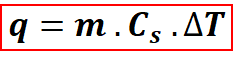# Problem: How much heat is required to warm 1.50 kg of sand from 26.0 oC to 100.0 oC?

###### FREE Expert Solution

The amount of heat required to warm a substance is determined using heat capacity. The equation below allows us to solve for the heat released or absorbed when warming a substance.For the equation above:

q = heat, J

m = mass (g)

Cs = specific heat capacity of sand (J / (g oC) )

ΔT= change in temperature. (°C)###### Problem Details

How much heat is required to warm 1.50 kg of sand from 26.0 oC to 100.0 oC?